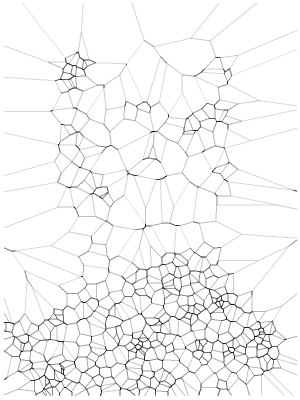# Frankenstein

March 7, 2017
By

Want to share your content on R-bloggers? click here if you have a blog, or here if you don't.

Remember me, remember me, but ah! forget my fate (Dido’s Lament, Henry Purcell)

A Voronoi diagram divides a plane based on a set of original points. Each polygon, or Voronoi cell, contains an original point and all that are closer to that point than any other.

This is a nice example of a Voronoi tesselation. You can find good explanations of Voronoi diagrams and Delaunay triangulations here (in English) or here (in Spanish).

A grayscale image is simply a matrix where darkness of pixel located in coordinates (i, j) is represented by the value of its corresponding element of the matrix: a grayscale image is a dataset. This is a Voronoi diagraman of Frankenstein:To do it I followed the next steps:

2. Convert it to gray scale
3. Turn it into a pure black and white image
4. Obtain a random sample of black pixels (previous image corresponds to a sample of 6.000 points)
5. Computes the Voronoi tesselation

Steps 1 to 3 were done with imager, a very appealing package to proccess and analice images. Step 5 was done with deldir, also a convenient package which computes Delaunay triangulation and the Dirichlet or Voronoi tessellations.

The next grid shows tesselations for sample size from 500 to 12.000 points and step equal to 500:

I gathered all previous images in this gif created with magick, another amazing package of R I discovered recently:This is the code:

```library(imager)
library(dplyr)
library(deldir)
library(ggplot2)
library(scales)

# Read and convert to grayscale

# This is just to define frame limits
x %>%
as.data.frame() %>%
group_by() %>%
summarize(xmin=min(x), xmax=max(x), ymin=min(y), ymax=max(y)) %>%
as.vector()->rw

# Filter image to convert it to bw
x %>%
threshold("45%") %>%
as.data.frame() -> df

# Function to compute and plot Voronoi tesselation depending on sample size
doPlot = function(n)
{
#Voronoi tesselation
df %>%
sample_n(n, weight=(1-value)) %>%
select(x,y) %>%
deldir(rw=rw, sort=TRUE) %>%
.\$dirsgs -> data

# This is just to add some alpha to lines depending on its longitude
data %>%
mutate(long=sqrt((x1-x2)^2+(y1-y2)^2),
alpha=findInterval(long, quantile(long, probs = seq(0, 1, length.out = 20)))/21)-> data

# A little bit of ggplot to plot results
data %>%
ggplot(aes(alpha=(1-alpha))) +
geom_segment(aes(x = x1, y = y1, xend = x2, yend = y2), color="black", lwd=1) +
scale_x_continuous(expand=c(0,0))+
scale_y_continuous(expand=c(0,0), trans=reverse_trans())+
theme(legend.position  = "none",
panel.background = element_rect(fill="white"),
axis.ticks       = element_blank(),
panel.grid       = element_blank(),
axis.title       = element_blank(),
axis.text        = element_blank())->plot

return(plot)
}

# I call the previous function and store resulting plot in jpeg format
i=5000
name=paste0("frankie",i,".jpeg")
jpeg(name, width = 600, height = 800, units = "px", quality = 100)
doPlot(i)
dev.off()

# Once all images are stored I can create gif
library(magick)
frames=c()
images=list.files(pattern="jpeg")

for (i in length(images):1)
{
x=image_scale(x, "300")
c(x, frames) -> frames
}
animation=image_animate(frames, fps = 2)
image_write(animation, "Frankenstein.gif")
```

R-bloggers.com offers daily e-mail updates about R news and tutorials about learning R and many other topics. Click here if you're looking to post or find an R/data-science job.
Want to share your content on R-bloggers? click here if you have a blog, or here if you don't.